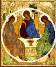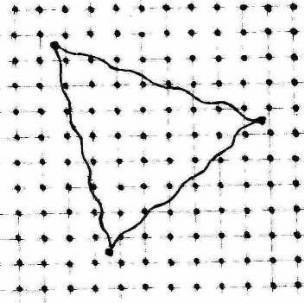REST MASS OF ELECTRON AND “CRYSTALLINE” STRUCTURE OF VACUUM. L.RegelsonFig.1. Nucleon in vacuum crystal
As Ervin Schroedinger asserted, the equation carrying his name, describes a real electronic wave field on which coulomb potential operates. Thus he has been compelled to refuse representation about electron as spatial localised indivisible particle.

One of arising questions: what is it – the rest mass for the wave field?

The simple answer to this question consists in the assumption: electron is plasmon. Differently,  the electronic field is excitation of plasmon type.

All basic properties of  wave field are defined by a dispersion relation between frequency and  wave length. Such relation for electronic field  (in the absence of potentials) is:

ω(2) с(2) к (2) = m(2) с(4) /ћ(2)

Where
ωo = 2π/λ – a wave vector.

The dispersion relation in the presence of potentials allows to deduce the wave equation which decisions satisfy to this relation. It is Dirac equation, in nonrelativistic approximation – Shroedinger equation. Calculation of group velocity by means of this relation leads to equations of relativistic dynamics for electronic wave packet.

The quantity

ωo = m(2) с(2) /ћ(2)
may be interpreted as plasma frequency of an electronic field.

However plasmon arises only in such medium in which collective fluctuations are possible. So, in a usual crystal, oscillation of electronic "cloud" of a crystal concerning  lattice nodes (plasma oscillation) and collective oscillation of  nodes concerning an electronic cloud (ionic-plasma oscillation) take place.

By analogy, plasmon character of an electronic field suggests the thought about the latent "crystal" structure of vacuum. One of possible models such «a vacuum crystal»: quarks as the nodes, connected by gluon "threads". In such "crystal" there can be various types of excitation, one of which is the electronic field.

If assume, that nodes of a vacuum crystal are presented by six types of quarks, arising subsystems can lead to six types plasmon excitation, according to six types of  leptons.

At once arises a question which the old theory of "aether" could not answer: why this medium does not offer resistance to movement of material bodies?

The most natural answer: the substance in a vacuum crystal possesses properties of superfluidity and superconductivity.

It may be presented, for example, in such a model: nucleons are the"defects" of a lattice, i.e. the nucleon consists of three quarks in  interstitial position (outside lattice nodes) jointed by gluon forces (Fig. 1). Such "a three" of quarks is similar to «Cooper pair» in usual superconductivity and  it moves through a vacuum crystal without resistance.

The hypothesis of vacuum or "world" crystal opens many various possibilities: for example, a simple explanation of gravitation. The cluster of "crystal defects" (i.e. a usual substance) should lead to distortions of a vacuum lattice that is equivalent to "a curvature of the space" or occurrence of gravitational forces.

Also it is possible to hope, that the conception of "a world crystal" will demonstrate its heuristic value in an explanation of such phenomena, as "a dark matter" and "dark energy".